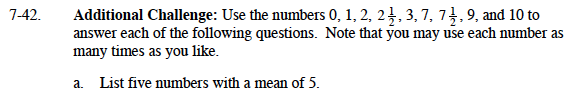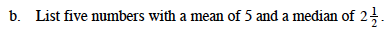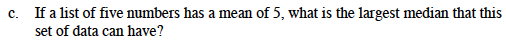### Home > MC1 > Chapter 7 > Lesson 7.2.1 > Problem7-42

7-42.If the mean is 5, and there are 5 numbers, what number do you want the sum of all of your numbers to be?Make sure that the desired median is the middle number when your numbers are in order.______, ______, ______, ______, ______

If the sum of the numbers must be 25, how can you arrange numbers so that the middle number is as high as possible?
Could the middle number be 10? Why or why not?